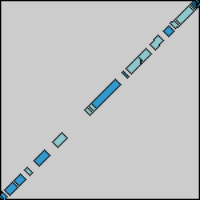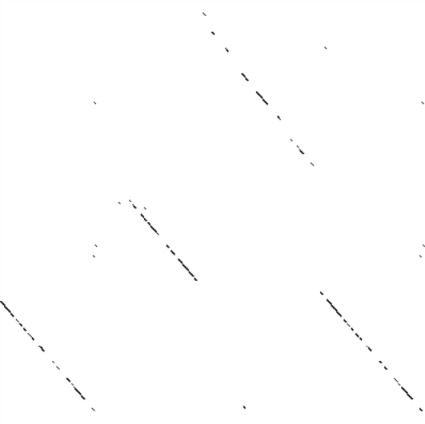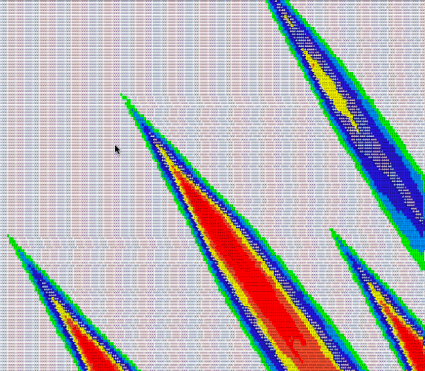home | about | contact | privacy statementIn the field of bioinformatics, one way to measure similarities between two (or more) sequences of DNA is to perform sequence alignment:
"a way of arranging the primary sequences of DNA, RNA, or protein to identify regions of similarity that may be a consequence of functional, structural, or evolutionary relationships between the sequences."
Think of it this way: you've got two random strands of DNA - how do you know where one starts and one begins? How do you know if they come from the same organism? A closely related pair? You might use sequence alignment to see how the two strands might line up in relation to each other - subsequences may indicate similar functionality, or conservation through evolution.

In "normal" programming terms, you've got a couple of strings and want to find out how you might align them so they they look as much like one another as possible.

There are plenty of ways to achieve that goal. Since we haven't done much programming on here lately, I thought it would be nice to focus on two very similar algorithms that do so: Needleman-Wunsch and Smith-Waterman.

The idea behind these two algorithms is that we have a scoring scheme we want to maximize as successive "matches" occur. One popular substitution matrix for scoring protein alignment is BLOSUM62 (and here's a good PDF describing how BLOSUM came about).

The particular scoring matrix you use will be determined by the goals you want to acheive. For our purposes, a simple matrix or two will suffice:

```@substitution_matrix =
[[" ", "a","c","g","t"],
["a",  1 , 0 , 0 , 0 ],
["c",  0 , 1 , 0 , 0 ],
["g",  0 , 0 , 1 , 0 ],
["t",  0 , 0 , 0 , 1 ]]

@substitution_matrix2 =
[[" ", "s", "e", "n", "d", "a"],
["s",  4 ,  0 ,  1 ,  0 ,  1 ],
["e",  0 ,  5 ,  0 ,  2 , -1 ],
["n",  1 ,  0 ,  6 ,  1 , -2 ],
["d",  0 ,  2 ,  1 ,  6 , -2 ],
["a",  1 , -1 , -2 , -2 ,  4 ]]
```

The first `@substitution_matrix` is fairly simplistic - give one point for each match, and ignore any mismatches or gaps introduced. In `@substitution_matrix2` what score should be given if "s" is aligned with "a"? (One.) What if "d" is aligned with another "d"? (Six.) The substitution matrix is simply a table telling you how to score particular characters when they are in the same position in two different strings.

After you've determined a scoring scheme, the algorithm starts scoring each pairwise alignment, adding to or subtracting from the overall score to determine which alignment should be returned. It uses dynamic programming, storing calculations in a table to avoid re-computation, which allows it to reverse course after creating the table to find and return the best alignment.

It feels strange to implement this as a class, but I did it to make it clear how trivially easy it is to derive Smith-Waterman (SW) from Needleman-Wunsch (NW). One design that jumps out at me would be to have a `SequenceAligner` where you can choose which algorithm as a method to run - then SW could use a NW algorithm where `min_score` is passed as a parameter to the method. Perhaps you can think of something even better.

Anyway, here's the Ruby class that implements the Needleman-Wunsch algorithm.

```class NeedlemanWunsch
@min_score = nil

def initialize(a, b, substitution_matrix, gap_penalty)
@a = a
@b = b

# convert to array if a/b were strings
@a = a.split("") if a.class == String
@b = b.split("") if b.class == String

@sm = substitution_matrix
@gp = gap_penalty
end

def get_best_alignment
construct_score_matrix
return extract_best_alignment_from_score_matrix
end

def construct_score_matrix
return if @score_matrix != nil #return if we've already calculated it

initialize_score_matrix

traverse_score_matrix do |i, j|
if i==0 && j==0
@score_matrix = 0
elsif i==0 #if this is a gap penalty square
@score_matrix[j] = j * @gp
elsif j==0 #if this is a gap penalty square
@score_matrix[i] = i * @gp
else
up = @score_matrix[i-1][j] + @gp
left = @score_matrix[i][j-1] + @gp
#@a and @b are off by 1 because we added cells for gaps in the matrix
diag = @score_matrix[i-1][j-1] + s(@a[i-1], @b[j-1])

max, how = diag, "D"
max, how = up, "U" if up > max
max, how = left, "L" if left > max

@score_matrix[i][j] = max
@score_matrix[i][j] = @min_score if @min_score != nil and max < @min_score

@traceback_matrix[i][j] = how
end
end
end

def extract_best_alignment_from_score_matrix
i = @score_matrix.length-1
j = @score_matrix.length-1
left = Array.new
top = Array.new
while i > 0 && j > 0
if @traceback_matrix[i][j] == "D"
left.push(@a[i-1])
top.push(@b[j-1])
i -= 1
j -= 1
elsif @traceback_matrix[i][j] == "L"
left.push "-"
top.push @b[j-1]
j -= 1
elsif @traceback_matrix[i][j] == "U"
left.push @a[i-1]
top.push "-"
i -= 1
else
puts "something strange happened" #this shouldn't happen
end
end
return left.join.upcase.reverse, top.join.upcase.reverse
end

def print_score_visualization
construct_score_matrix
print_as_table(@score_matrix)
end

def print_traceback_matrix
construct_score_matrix
print_as_table(@traceback_matrix)
end

def print_as_table(the_matrix)
puts
puts "a=" + @a.to_s
puts "b=" + @b.to_s
puts
print "   "

@b.each_index {|elem| print " " + @b[elem].to_s }

puts ""
traverse_score_matrix do |i, j|
if j==0 and i > 0
print @a[i-1]
elsif j==0
print " "
end
print " " + the_matrix[i][j].to_s

puts "" if j==the_matrix[i].length-1
end
end

def traverse_score_matrix
@score_matrix.each_index do |i|
@score_matrix[i].each_index do |j|
yield(i, j)
end
end
end

def initialize_score_matrix
@score_matrix = Array.new(@a.length+1)
@traceback_matrix = Array.new(@a.length+1)
@score_matrix.each_index do |i|
@score_matrix[i] = Array.new(@b.length+1)
@traceback_matrix[i] = Array.new(@b.length+1)
@traceback_matrix.each_index {|j| @traceback_matrix[j] = "L" if j!=0 }
end

@traceback_matrix.each_index {|k| @traceback_matrix[k] = "U" if k!=0 }
@traceback_matrix = "f"
end

def s(a, b) #check the score for bases a. b being aligned
for i in 0..(@sm.length-1)
break if a.downcase == @sm[i].downcase
end

for j in 0..(@sm.length-1)
break if b.downcase == @sm[j].downcase
end
return @sm[i][j]
end
end

```

Needleman-Wunsch follows that path, and finds the best global alignment possible. Smith-Waterman truncates all negative scores to 0, with the idea being that as the alignment score gets smaller, the local alignment has come to an end. Thus, it's best to view it as a matrix, perhaps with some coloring to help you visualize the local alignments.

All we really need to get Smith-Waterman from our implementation of Needleman-Wunsch above is this:

```class SmithWaterman < NeedlemanWunsch
def initialize(a, b, substitution_matrix, gap_penalty)
@min_score = 0
super(a, b, substitution_matrix, gap_penalty)
end
end
```

However, it would be nice to be able to get a visualization matrix. This matrix should be able to use windows of pairs instead of each and every pair, since there can be thousands or millions or billions of base pairs we're aligning. Let's add a couple of methods to that effect:

```#modify array class to include extract_submatrix method
class Array
def extract_submatrix(row_range, col_range)
self[row_range].transpose[col_range].transpose
end
end

require 'needleman-wunsch'
class SmithWaterman < NeedlemanWunsch
def initialize(a, b, substitution_matrix, gap_penalty)
@min_score = 0
super(a, b, substitution_matrix, gap_penalty)
end

def print_score_visualization(window_size=nil)
return super() if window_size == nil

construct_score_matrix

#score_matrix base indexes
si = 1

#windowed_matrix indexes
wi = 0

windowed_matrix = initialize_windowed_matrix(window_size)

#compute the windows
while (si < @score_matrix.length)
sj = 1
wj = 0
imax = si + window_size-1
imax = @score_matrix.length-1 if imax >= @score_matrix.length

while (sj < @score_matrix.length)
jmax = sj + window_size-1
jmax = @score_matrix.length-1 if jmax >= @score_matrix.length

current_window = @score_matrix.extract_submatrix(si..imax, sj..jmax)
current_window_score = 0
current_window.flatten.each {|elem| current_window_score += elem}

begin
windowed_matrix[wi][wj] = current_window_score
rescue
end

wj += 1
sj += window_size
end

wi += 1
si += window_size
end

#find max score of windowed_matrix
max_score = 0
windowed_matrix.flatten.each{|elem| max_score = elem if elem > max_score}

max_score += 1 #so the max normalized score will be 9 and line up properly

#normalize the windowed matrix to have scores 0-9 relative to percent of max_score
windowed_matrix.each_index do |i|
windowed_matrix[i].each_index do |j|
begin
normalized_score = windowed_matrix[i][j].to_f / max_score * 10

windowed_matrix[i][j] = normalized_score.to_i
rescue

end
end
end

#print the windowed matrix
windowed_matrix.each_index do |i|
windowed_matrix[i].each_index do |j|
print windowed_matrix[i][j].to_s
end
puts
end
end

def initialize_windowed_matrix(window_size)
windowed_matrix = Array.new(((@a.length+1).to_f)/window_size)
windowed_matrix.each_index do |i|
windowed_matrix[i] = Array.new(((@b.length+1).to_f)/window_size)
end
return windowed_matrix
end
end
```

And now we'll try it out. First, we take two sequences and perform a DNA dotplot analysis on them:Then, we can take our own visualization, do a search and replace to colorize the results by score, and have a look:Lo and behold, they look quite similar!

I understand the algorithms are a bit complex and particularly well explained, so I invite questions about them in particular. As always, comments and (constructive) criticisms are encouraged as well.

Hey! Why don't you make your life easier and subscribe to the full post or short blurb RSS feed? I'm so confident you'll love my smelly pasta plate wisdom that I'm offering a no-strings-attached, lifetime money back guarantee!

Let me make a request for help and a quick announcement, and then I'll get you back to your regularly scheduled on-topic reading:

I need a good C/C++ IDE
I've been doing a lot of work in C++ lately for bioinformatics, and DevC++ is just not going to make the cut. My friend Michael suggested I use Visual Studio, but I thought I'd throw this out there and see what everyone else thought and try out a few more.

I'd like it to work on Windows, but I wouldn't mind hearing some Mac choices for the fun of it. Ideally, it would have a lot of the features of IntelliJ IDEA, but if it's not that awesome, I could probably get by. DevC++ is just broken for me. I won't go into too much detail, as I think those guys are providing a good service and I'm not helping them out myself, but sometimes headers get that do long has been the least of my troubles.

I'd like to know of both free and paid versions.

I've finally started using my twitter account. I started the account a while back, but never really "got it." I guess the other day the light bulb went off in my head. It's like email + IRC + instant messaging + blogging all in one.

Anyway, if you're on twitter and want to start following me, I'll get notified and probably start following you as well. Of course, if I start getting too many updates, I'll randomly stop following some people. Try not to take it personally if that happens.

I try not to give the minute details of my life like "I just woke up" or "I'm voting for so-and-so." Instead, I've been trying to stay on topic of this blog (programming and technology), but with small thoughts about whatever I happen to be working on. Of course, you'll find some responses to other people won't always be on my main topic.

I hope to see you on there. And if you can help in the C++ IDE department, please let me know!Web CodeOdor.com

Topics
.NET (19)
AI/Machine Learning (14)
Answers To 100 Interview Questions (10)
Bioinformatics (2)
C and Cplusplus (6)
cfrails (22)
ColdFusion (78)
Customer Relations (15)
Databases (3)
DRY (18)
DSLs (11)
Future Tech (5)
Games (5)
Groovy/Grails (8)
Hardware (1)
IDEs (9)
Java (38)
JavaScript (4)
Linux (2)
Lisp (1)
Mac OS (4)
Management (15)
MediaServerX (1)
Miscellany (76)
Productivity (11)
Programming (168)
Programming Quotables (9)
Rails (31)
Ruby (67)
scriptaGulous (4)
Software Development Process (23)
TDD (41)
TDDing xorblog (6)
Tools (5)
Web Development (8)
Windows (1)
With (1)
YAGNI (10)

Resources
Agile Manifesto & Principles
Principles Of OOD
ColdFusion
CFUnit
Ruby
Ruby on Rails
JUnit

 RSS 2.0: Full Post | Short Blurb Subscribe by email: Delivered by FeedBurner